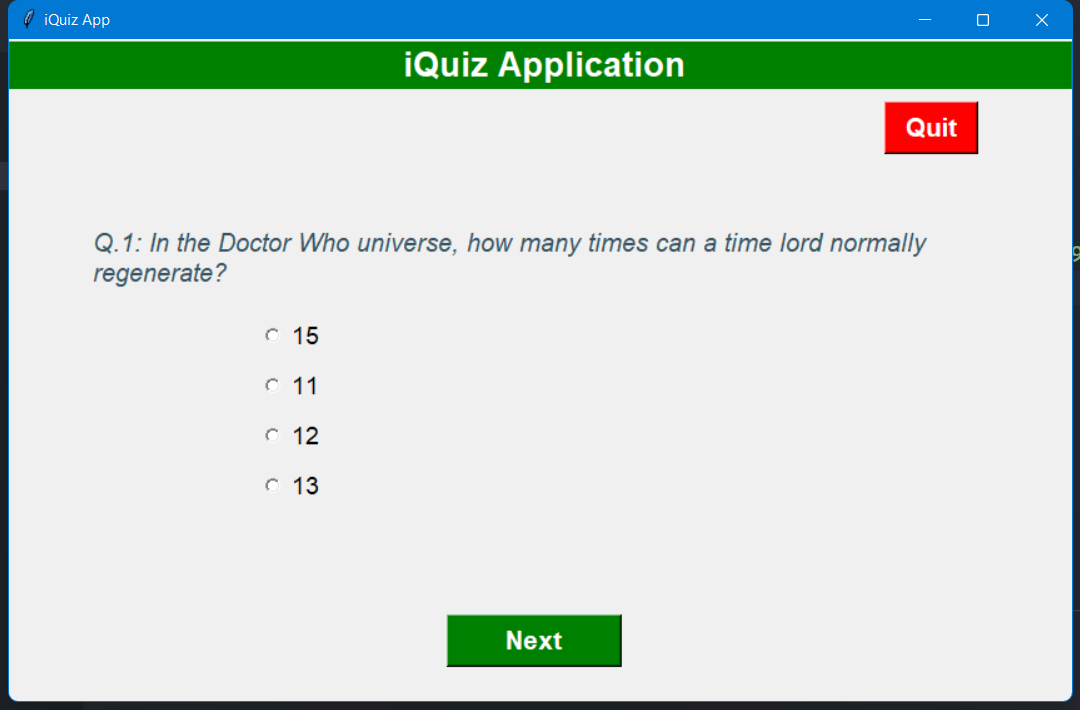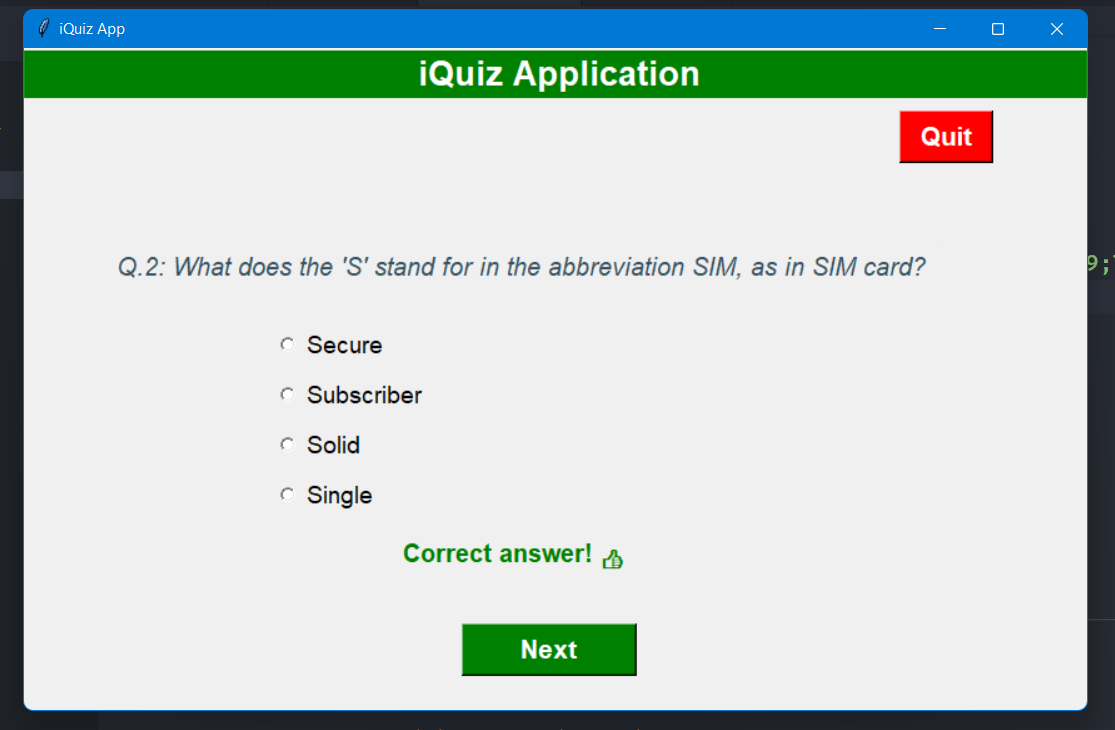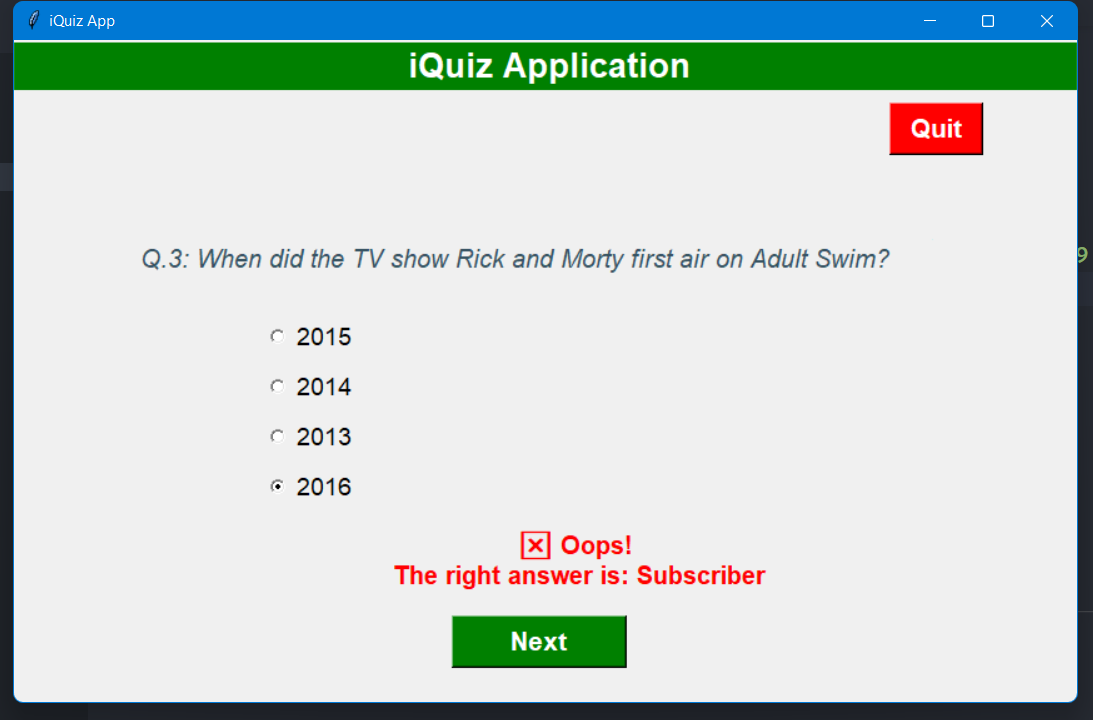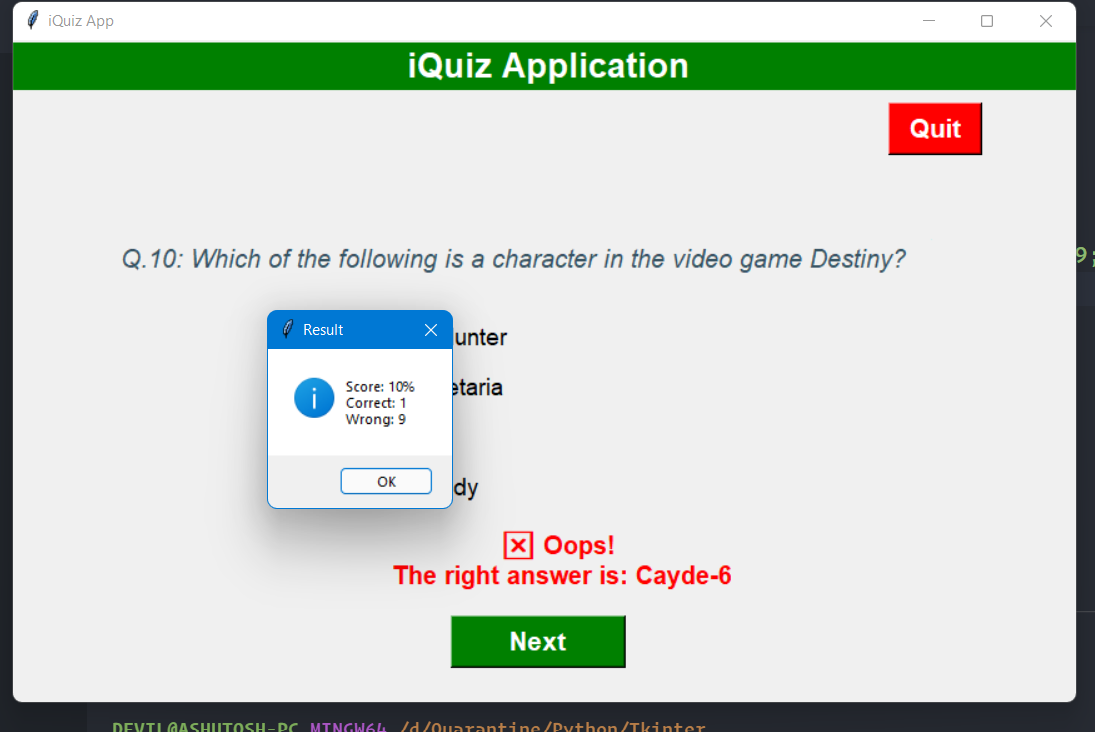# GUI Quiz Application using Tkinter and Open Trivia DB

ProgrammingIn this blog, we'll learn to build a Graphical User Interface (GUI) Quiz Application using the Tkinter Python built-in module. The task is to ask multiple-choice questions, collect user answers and finally display the results. Before coding the GUI, we'll first see how to fetch multiple-choice questions, their correct answers, and the choices from the Open Trivia DB API. The Open Trivia Database provides a completely free JSON API for use in programming projects. Use of this API does not require an API Key. To make the task more interesting, we'll also randomize the order of choices.

The following modules and concepts will be utilized in this project:

We'll be segregating our different functionalities into different classes and files.

# Fetching Questions

As we discussed above, we'll be using the Open Trivia DB API to get the questions. Head over to their API, select the number of questions, category, and difficulty. The question type should be Multiple Choice and the encoding should be Default Encoding. Click on Generate API URL and you'll get an API URL.

Sample API URL: `https://opentdb.com/api.php?amount=10&type=multiple`

For fetching the questions, we'll be using `requests` module. It can be installed as:

``\$ pip install requests``

Let's create a Python file `quiz_data.py` to fetch the quiz questions and answers using the API URL generated above.

``````import requests

parameters = {
"amount": 10,
"type": "multiple"
}

response = requests.get(url="https://opentdb.com/api.php", params=parameters)
question_data = response.json()["results"]``````

In the above script, instead of directly adding the `amount` and `type` parameters in the URL, we have created a `parameters` dictionary and added the respective values. After that, we're making a GET request using requests library on the Open Trivia DB API URL. A sample JSON response looks like this:

``````{
"response_code": 0,
"results": [
{
"category": "Entertainment: Video Games",
"type": "multiple",
"difficulty": "hard",
"question": "What was the name of the hero in the 80s animated video game &#039;Dragon&#039;s Lair&#039;?",
"incorrect_answers": ["Arthur", "Sir Toby Belch", "Guy of Gisbourne"]
},
{
"category": "Entertainment: Video Games",
"type": "multiple",
"difficulty": "medium",
"question": "Which of these game franchises were made by Namco?",
"incorrect_answers": ["Street Fighter", "Mortal Kombat", "Dragon Quest"]
}
]
}
``````

The JSON data contains a dictionary with two keys - `response_code` and `results`. The `response_code` tells developers what the API is doing. The `results` is a list we are interested in. So, we have stored the value of results in a variable `question_data`.

# Question Model

Question model is nothing but a Python class with three attributes - `question_text`, `correct_answer` and `choices`. question_text is the question, correct_answer is the correct answer for that question and choices is a list of options for that question.

Let's create a `question_model.py` file and create the class in it:

``````class Question:
def __init__(self, question: str, correct_answer: str, choices: list):
self.question_text = question
self.choices = choices
``````

# Quiz Brain

The `QuizBrain`, as the name suggests, is the brain of the application. Let's create `quiz_brain.py` file and add the following code there:

``````class QuizBrain:

def __init__(self, questions):
self.question_no = 0
self.score = 0
self.questions = questions
self.current_question = None

def has_more_questions(self):
"""To check if the quiz has more questions"""

return self.question_no < len(self.questions)

def next_question(self):
"""Get the next question by incrementing the question number"""

self.current_question = self.questions[self.question_no]
self.question_no += 1
q_text = self.current_question.question_text
return f"Q.{self.question_no}: {q_text}"

"""Check the user answer against the correct answer and maintain the score"""

self.score += 1
return True
else:
return False

def get_score(self):

wrong = self.question_no - self.score
score_percent = int(self.score / self.question_no * 100)
return (self.score, wrong, score_percent)
``````

The `QuizBrain` class takes `questions`, list of questions. Additionally, the `question_no` and `score` attributes are initialized with `0` and the `current_question` is set to `None` initially.

The first method `has_more_questions()` checks whether the quiz has more questions or not. The next method `next_question()` gets the question from the `questions` list at index `question_no` and then increments the `question_no` attribute returns a formatted question. The `check_answer()` method takes `user_answer` as an argument and checks whether the user's answer is correct or not. It also maintains the score and returns boolean values. The last method `get_score()` returns the number of correct answers, wrong answers, and score percentage.

# Quiz UI

Let's move to the next part where we create the user interface of the application. Create a `quiz_ui.py` file and add the following content:

``````from tkinter import Tk, Canvas, StringVar, Label, Radiobutton, Button, messagebox
from quiz_brain import QuizBrain

THEME_COLOR = "#375362"

class QuizInterface:

def __init__(self, quiz_brain: QuizBrain) -> None:
self.quiz = quiz_brain
self.window = Tk()
self.window.title("iQuiz App")
self.window.geometry("850x530")

# Display Title
self.display_title()

# Creating a canvas for question text, and dsiplay question
self.canvas = Canvas(width=800, height=250)
self.question_text = self.canvas.create_text(400, 125,
text="Question here",
width=680,
fill=THEME_COLOR,
font=(
'Ariel', 15, 'italic')
)
self.display_question()

# Declare a StringVar to store user's answer

self.display_options()

# To show whether the answer is correct or wrong
self.feedback = Label(self.window, pady=10, font=("ariel", 15, "bold"))
self.feedback.place(x=300, y=380)

# Next and Quit Button
self.buttons()

# Mainloop
self.window.mainloop()

def display_title(self):
"""To display title"""

# Title
title = Label(self.window, text="iQuiz Application",
width=50, bg="green", fg="white", font=("ariel", 20, "bold"))

# place of the title
title.place(x=0, y=2)

def display_question(self):
"""To display the question"""

q_text = self.quiz.next_question()
self.canvas.itemconfig(self.question_text, text=q_text)

"""To create four options (radio buttons)"""

# initialize the list with an empty list of options
choice_list = []

# position of the first option
y_pos = 220

# adding the options to the list
while len(choice_list) < 4:

# setting the radio button properties
value='', font=("ariel", 14))

# adding the button to the list

# placing the button

# incrementing the y-axis position by 40
y_pos += 40

return choice_list

def display_options(self):
"""To display four options"""

val = 0

# deselecting the options

# looping over the options to be displayed for the
# text of the radio buttons.
for option in self.quiz.current_question.choices:
self.opts[val]['text'] = option
self.opts[val]['value'] = option
val += 1

def next_btn(self):
"""To show feedback for each answer and keep checking for more questions"""

# Check if the answer is correct
self.feedback["fg"] = "green"
else:
self.feedback['fg'] = 'red'
self.feedback['text'] = ('\u274E Oops! \n'

if self.quiz.has_more_questions():
# Moves to next to display next question and its options
self.display_question()
self.display_options()
else:
# if no more questions, then it displays the score
self.display_result()

# destroys the self.window
self.window.destroy()

def buttons(self):
"""To show next button and quit button"""

# The first button is the Next button to move to the
# next Question
next_button = Button(self.window, text="Next", command=self.next_btn,
width=10, bg="green", fg="white", font=("ariel", 16, "bold"))

# palcing the button  on the screen
next_button.place(x=350, y=460)

# This is the second button which is used to Quit the self.window
quit_button = Button(self.window, text="Quit", command=self.window.destroy,
width=5, bg="red", fg="white", font=("ariel", 16, " bold"))

# placing the Quit button on the screen
quit_button.place(x=700, y=50)

def display_result(self):
"""To display the result using messagebox"""
correct, wrong, score_percent = self.quiz.get_score()

correct = f"Correct: {correct}"
wrong = f"Wrong: {wrong}"

# calculates the percentage of correct answers
result = f"Score: {score_percent}%"

# Shows a message box to display the result
messagebox.showinfo("Result", f"{result}\n{correct}\n{wrong}")
``````

Well, a very large script but if you look at it carefully, it's quite easy to understand. The code is well documented.

# Getting Things Together

Since all the components are ready to be integrated together, let's create a `main.py` file and add the following content there:

``````from question_model import Question
from quiz_data import question_data
from quiz_brain import QuizBrain
from quiz_ui import QuizInterface
from random import shuffle
import html

question_bank = []
for question in question_data:
choices = []
question_text = html.unescape(question["question"])
choices.append(html.unescape(ans))
shuffle(choices)
question_bank.append(new_question)

quiz = QuizBrain(question_bank)

quiz_ui = QuizInterface(quiz)

print("You've completed the quiz")
``````

Let's import all the classes from the different files we have created. In addition to that, we also need `shuffle` method from the `random` module and the `html` module. We have a list `question_bank`. We are iterating over the `question_data` that we receive from `quiz_data.py` file. If you see the sample response, you will find some text such as `&#039;Dragon&#039`. They need to be unescaped using the `html.unescape` method. We have a `choices` list that will contain the correct answer as well as the incorrect answers. The list will be shuffled using the `shuffle` method from the `random` module. After shuffling, we are creating question using the `Question` model from `quiz_model.py` file and appending it the `question_bank` list.

Next, we're creating an object `quiz` of QuizBrain class which requires a list of questions. So, we're passing `question_bank` to it. After that, we're creating an object `quiz_ui` of QuizInterface class which requires an object of QuizBrain class, so we have passed the newly created `quiz` object to it.

Now that everything is ready, we are ready to run the application.

``\$ python main.py``

### Screenshots# Conclusion

Hope you liked the quiz application. You can add more features and make the UI more attractive if you wish to.

Code Repository: https://github.com/ashutoshkrris/GUI-Quiz-Tkinter